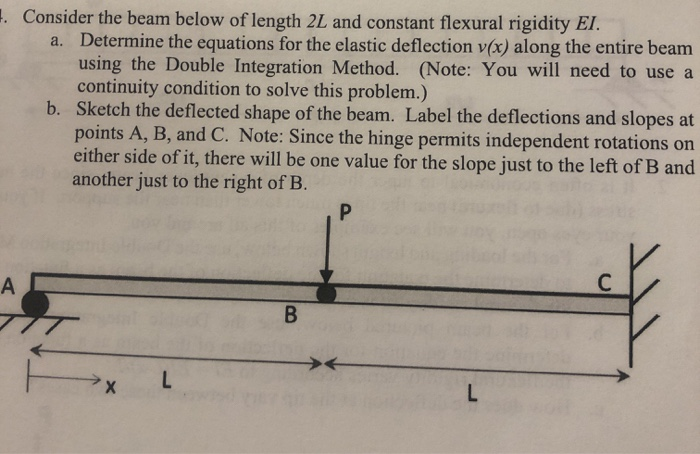## Blogcourseexpert
February 12, 2020. Consider the beam below of length 2L and constant flexural rigidity El. a. Determine the equations for the elastic deflection v(x) along the entire beam using the Double Integration Method. (Note: You will need to use a continuity condition to solve this problem.) b. Sketch the deflected shape of the beam. Label the deflections and slopes at points A, B, and C. Note: Since the hinge permits independent rotations on either side of it, there will be one value for the slope just to the left of B and another just to the right of B. P C A В X